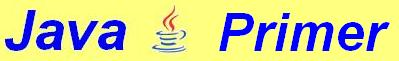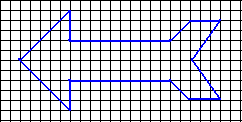13. Drawing in an applet (2)

In chapter 2 of this tutorial, we already came across a few figures that Java allows us to draw:

 Line: Rectangle: Ellipse: Circle:

Of course, if we want to draw a circle, h and b must be equal. In the example, we call them d (diameter).

We have seen that rectangles and ellipses can be filled up with these statements:

g.fillRect (x, y, b, h);
g.fillOval (x, y, b, h);

Drawing in color
If you want to draw in color, you must first set the color with:

g.setColor (Color.red);

Of course, red is not the only color available. The class Color contains the following pre-defined colors:

 Color.black Color.green Color.pink Color.blue Color.lightGray Color.red Color.cyan Color.magenta Color.white Color.darkGray Color.orange Color.yellow Color.gray

Thus we can make colored figures like these:

It is also possible to draw rounded rectangles with the functions drawRoundRect and fillRoundRect. As you can see, we need two extra arguments for the degree of rounding.

'Raised' figures
Java can also draw rectangles that suggest their surface is raised (or lowered). For that purpose, java has the method fill3DRect. This method has a fifth argument of the boolean type, which allows us to draw a a dark rectangle with a light outline or the other way round.

Both are shown below. Just for comparison, we shall add a 'normal' rectangle on the right.

The basic colors red, green and blue can be mixed in any quantities we want. This can be done like this:

int red=60, green=10, blue=25;
Color myColor = new Color (red, green, blue);
g.setColor (myColor);
g.drawString ("Is this written in purple?", 10, 100);

The values of red, green and blue must be in the range of 0 to 255.

We can make an applet with three textfields into which the values for red, green and blue can be input. In order to make things a little more efficient, we'll use a few objects in the form of arrays consisting of three elements (0, 1, 2).

// Mixing color by means of three numbers (RGB)
import java.applet.*;
import java.awt.*;
public class Colors extends Applet {
boolean wrong;
// rgb through rgb: which contain the
// numerical values for red, green and blue.
int rgb[ ] = new int;
TextField box[ ] = new TextField;
// The labels text through text label the
// input boxes with the names of the colors.
Label text[ ] = new Label;
String colors[ ] = {"red:", "green:", "blue:"};
Button button = new Button ("show");

public void init () {
for (int i=0; i<3; i++) {
box[i] = new TextField("0");
text[i] = new Label(colors[i]);
}
}

public void paint (Graphics g) {
// Now a dark rectangle is drawn
// which should suggest a shadow.
g.setColor (Color.darkGray);
g.fillRect (15,15,400,240);
g.drawRect (0,0,385,225);
g.setColor (Color.white);
g.fillRect (0,0,385,225);
g.setColor (Color.black);
if (wrong)
g.drawString ("Only 0 to 255!", 100, 100);
else {
Color color=new Color (rgb,rgb,rgb);
g.setColor (color);
g.fillRect (100,50,200,130);
}
}

int toByte (String s) {
// returns -1 if string cannot be converted
// to an integer in the range of 0 to 255.
int length = s.length();
s = s.trim(); // removes any trailing spaces
if (length > 3)
return -1;
for (int i=0; i<length; i++) {
char c = s.charAt(i);
if (c<'0' || c>'9')
return -1;
}
int number = Integer.parseInt(s);
if (number > 255)
return -1;
else
return number;
}

public boolean action (Event e, Object o) {
if (e.target==button) {
wrong = false;
for (int i=0; i<3; i++) {
String temp = box[i].getText();
rgb[i] = toByte(temp);
if (rgb[i] == -1)
wrong = true;
}
}
repaint();
return true;
}

}

Our home-made method toByte converts the string (which has been typed into the textfield) into a number. If this does not yield a number in the range of 0 to 255, the value -1 is returned.

This is what the applet looks like:

Special shapes
In Java, it is possible to draw figures that would be hard to make with lines, rectangles and ellipses.

Suppose we want to draw an arrow like the one shown here:If we want to make this figure, we would have to connect twelve points.

We can draw this figure by making use of the method drawPolygon. This method requires three arguments: an array of x-coordinates and an array of y-coordinates. The third argument is the length of either of the arrays (which are equal in size, of course).

The method paint which can draw this arrow looks like this:
public void paint (Graphics g) {
// array h for horizontal displacements:
int h[ ] = {10,70,70,170,190,220,
190,220,190,170,70,70,10};
// array v for vertical displacements:
int v[ ] = {60,10,40,40,20,20,
60,100,100,80,80,110,60};
g.drawPolygon (h, v, h.length);
}
Apart from the method drawPolygon, there is fillPoygon, which makes figures like the one shown here:

Passing arguments to an applet
If you wish to do so, you can pass arguments to an applet from within the HTML file.

Twelve of the figures shown in this page are made by the same applet named FiguurDemo.class, which is launched each time with different arguments.

In the HTML file that makes this page, the applet to draw the yellow rectangle is launched like this:
<applet code = FiguurDemo.class
width = 320  height = 240>
param name = "figure" value = "5">
</applet>
The statement param name is used to assign a name under which the argument can be imported into the applet. Mind that only strings can be used as arguments, in this case the string "5".

The string is imported into the applet (usually in the method init). In the applet FiguurDemo.java, this takes place in the following way:

String tmp = getParameter ("figure");

After this line, the String tmp will contain the value "5" (or another value that was assigned to "figure" in the html file.

Converting this string into a number could, for instance, be done like this:

int figure = Integer.parseInt(tmp);

Let us have a look at a program in which a few arguments are passed from the html file to the applet.

We shall pass background color, font color and font size as arguments. The HTML file will look like this:
<applet code=ParamDemo.class
width=320 height=240>
<PARAM NAME=fontColor VALUE="yellow">
<PARAM NAME=backgrColor VALUE="blue">
<PARAM NAME=fontSize VALUE="32">
</applet>
All these values are imported into the applet. To prevent the applet from crashing if faulty (or no) arguments are passed, a few error checks have been implemented.
// Demonstration of parameters
// that are passed from the HTML file.
import java.applet.*;
import java.awt.*;
public class ParamDemo extends Applet {
String fontColor;

public void init () {
fontColor = getParameter("fontColor");
String backgrColor = getParameter("backgrColor");
if (backgrColor != null) {
if (backgrColor.equals("blue"))
setBackground (Color.blue);
else if (backgrColor.equals("green"))
setBackground (Color.green);
else setBackground (Color.lightGray);
}
}

public void paint (Graphics g) {
if (fontColor != null) {
if (fontColor.equals("yellow"))
g.setColor (Color.yellow);
else if (fontColor.equals("orange"))
g.setColor (Color.orange);
else g.setColor (Color.black);
}
int fSize = 15;
try {
fSize = Integer.parseInt(getParameter("fontSize"));
}
catch (java.lang.NumberFormatException e) { }
g.setFont(new Font ("Helvetica", Font.BOLD, letgr));
g.drawString ("ParameterDemo", 20, 100);
}
}
In this applet, two error checks are carried out:
• A string that has not been assigned any value contains nothing. In Java, that's referred to as the object null. In the case of the two color strings, we check whether the string has actually been assigned a value. If not, the value will be equal to null.
• A string can be converted to int with the function parseInt (which is part of the class Integer. The method parseInt does not check whether the string can actually yield a valid integer. That is why we catch any errors with a  try-catch construction. We don't write anything between the curly braces because fSize has been assigned the value 15. If no new value is assigned, the value will just remain 15.

Exercise 13.1
Write an applet with two textfields and a button. After inputting width and height and clicking the button, a rectangle with the desired width and height will be drawn.

Exercise 13.2
The same applet as 13.1. Make the applet issue an error warning if strings have been input that cannot be turned into positive integers or if the values are too large to fit into the applet window (irrespective of the size defined in the html file).

Exercise 13.3
Make an applet that draws a rectangle whose height, width and filling color are determined by arguments laid down in the html file. The applet should issue a warning if the arguments are incorrect. It must also signal if the height and/or width are either negative or too large to allow the rectangle to fit into the applet window.

(c) 2005, Thomas J.H. Luif